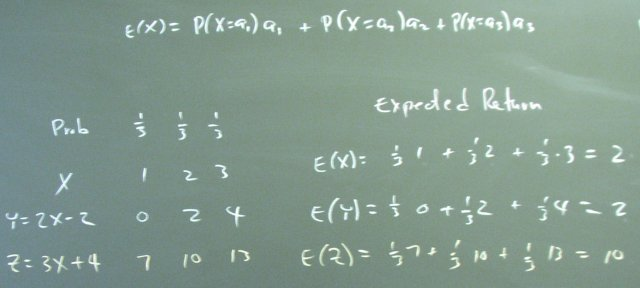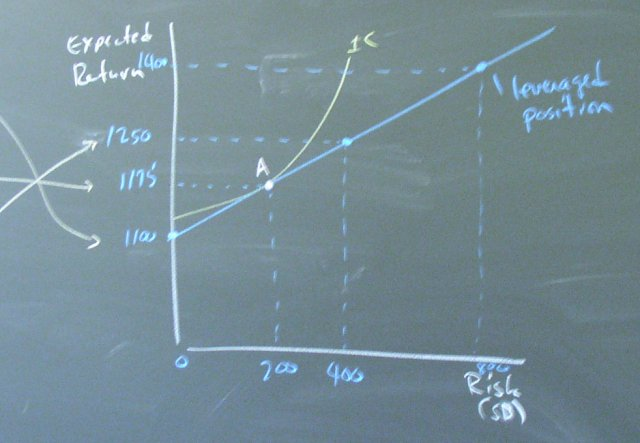## March 20, 2003

### Help with Homework 3

Questions 1 and 4 develop the budget constraint for our diargram of risk vs. expected return using the following mathematical results.Question 1 attempts to help you understand the "aX+b" rules using arithmetic calculations instead of a symbolic proof.Question 4 applies these results to analyzing the risk and expected return for various portfolios.Posted by bparke at March 20, 2003 02:38 PM